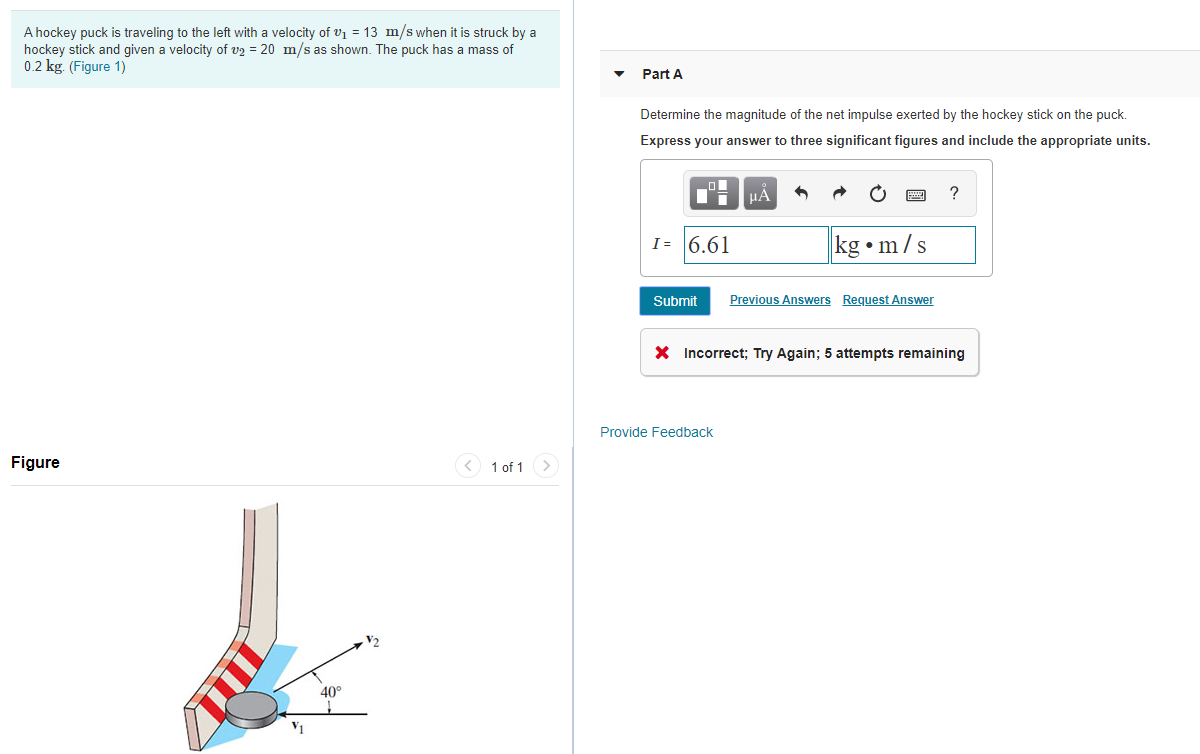# A hockey puck is traveling to the left with a velocity of v1 = 13 m/s when it is struck by ahockey stick and given a velocity of v2 20 m/s as shown. The puck has a mass of0.2 kg. (Figure 1)Part ADetermine the magnitude of the net impulse exerted by the hockey stick on the puck.Express your answer to three significant figures and include the appropriate units.?HALiI=6.61kg m/sSubmitPrevious AnswersRequest AnswerIncorrect; Try Again; 5 attempts remainingProvide Feed backFigure1 of 1V240°

Questionhelp_outlineImage TranscriptioncloseA hockey puck is traveling to the left with a velocity of v1 = 13 m/s when it is struck by a hockey stick and given a velocity of v2 20 m/s as shown. The puck has a mass of 0.2 kg. (Figure 1) Part A Determine the magnitude of the net impulse exerted by the hockey stick on the puck. Express your answer to three significant figures and include the appropriate units. ? HA Li I=6.61 kg m/s Submit Previous Answers Request Answer Incorrect; Try Again; 5 attempts remaining Provide Feed back Figure 1 of 1 V2 40° fullscreen
check_circleExpert Solution
Step 1

The initial velocity is v1= -13i m/s

The final velocity is v2 =(20cos40)i+(20sin40)j

= 15.3i+12.85j m/s

Step 2

The equation for impu...

### Want to see the full answer?

See Solution

#### Want to see this answer and more?

Solutions are written by subject experts who are available 24/7. Questions are typically answered within 1 hour*

See Solution
*Response times may vary by subject and question
Tagged in

### Physics